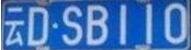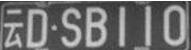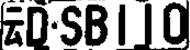# Python+OpenCV实现车牌字符分割和识别

更新时间：2018年03月31日 09:03:51   作者：Jdfohewk我要评论

1、图像处理

①将图片灰度化

②将灰度图片二值化

③旋转调平

④去燥

2、图像切割和识别

①图像切割

a.将每一列的1值和0值分别统计起来。

b.根据每一列的0-1总和变换来切割字符

②图像识别

a.逐个像素比对，如果一致则count加一，最后根据count值确定匹配结果。

b.投影匹配：将每行、每列的像素位统计起来，根据差值大小来确定匹配结果。

```import cv2

# 1、读取图像，并把图像转换为灰度图像并显示
img_gray = cv2.cvtColor(img, cv2.COLOR_BGR2GRAY) # 转换了灰度化
cv2.imshow('gray', img_gray) # 显示图片
cv2.waitKey(0)

# 2、将灰度图像二值化，设定阈值是100
img_thre = img_gray
cv2.threshold(img_gray, 100, 255, cv2.THRESH_BINARY_INV, img_thre)
cv2.imshow('threshold', img_thre)
cv2.waitKey(0)

# 3、保存黑白图片
cv2.imwrite('thre_res.png', img_thre)

# 4、分割字符
white = [] # 记录每一列的白色像素总和
black = [] # ..........黑色.......
height = img_thre.shape
width = img_thre.shape
white_max = 0
black_max = 0
# 计算每一列的黑白色像素总和
for i in range(width):
s = 0 # 这一列白色总数
t = 0 # 这一列黑色总数
for j in range(height):
if img_thre[j][i] == 255:
s += 1
if img_thre[j][i] == 0:
t += 1
white_max = max(white_max, s)
black_max = max(black_max, t)
white.append(s)
black.append(t)
print(s)
print(t)

arg = False # False表示白底黑字；True表示黑底白字
if black_max > white_max:
arg = True

# 分割图像
def find_end(start_):
end_ = start_+1
for m in range(start_+1, width-1):
if (black[m] if arg else white[m]) > (0.95 * black_max if arg else 0.95 * white_max): # 0.95这个参数请多调整，对应下面的0.05
end_ = m
break
return end_

n = 1
start = 1
end = 2
while n < width-2:
n += 1
if (white[n] if arg else black[n]) > (0.05 * white_max if arg else 0.05 * black_max):
# 上面这些判断用来辨别是白底黑字还是黑底白字
# 0.05这个参数请多调整，对应上面的0.95
start = n
end = find_end(start)
n = end
if end-start > 5:
cj = img_thre[1:height, start:end]
cv2.imshow('caijian', cj)
cv2.waitKey(0) ```